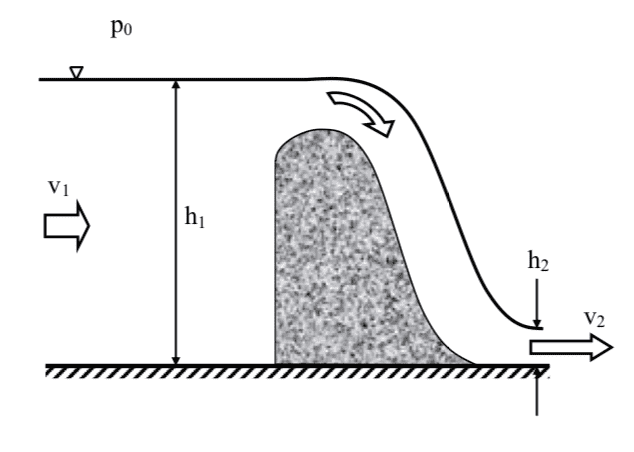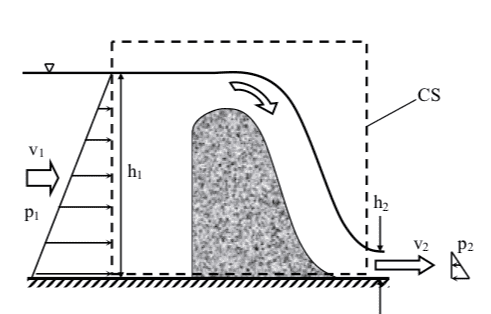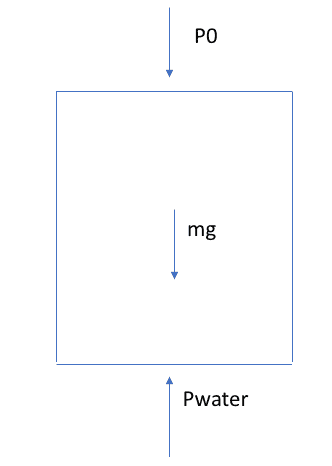# Control Volume, Fluid Mechanics

## Homework Statement

Water flows over a dam as depicted below. Across section 1 and 2 we have a hydrostatic pressure distribution. Calculate the force on the dam.## The Attempt at a Solution

My question is regarding control volume. The solution manual has given us this:The two hydrostatic forces are understandable, as well as an unknown force Fk inside the CV. But what about the pressure acting on top and at the bottom of the CV? At the top the pressure must be P0, but what about at the bottom? Shouldn't this be an unknown pressure from the water? And when do we have to consider the weight of the fluid, i.e. mg in our CV analyses?

#### Attachments

Last edited:

Chestermiller
Mentor
The control volume analysis for this kind of problem typically focuses only on the horizontal direction, and the vertical pressure forces acting on the top and bottom do not affect the horizontal force balance (remember, force is a vector). If you apply the macroscopic momentum balance to the control volume for the vertical direction, then you get the weight of the water.

Thank you, Chestermiller. Really appreciate your help here!

Would this be the forces in the vertical direction? For the same CV as above (I reckon I might miss a contact force from the dam?)I also assume these would have to sum to zero as we only have flow in the horizontal direction (using sigmaF = rho*q*v,out - rho*q*v,in = 0)

#### Attachments

Chestermiller
Mentor
Thank you, Chestermiller. Really appreciate your help here!

Would this be the forces in the vertical direction? For the same CV as above (I reckon I might miss a contact force from the dam?)

View attachment 225974

I also assume these would have to sum to zero as we only have flow in the horizontal direction (using sigmaF = rho*q*v,out - rho*q*v,in = 0)
The macroscopic momentum balance in the vertical direction is F= mg, where F is the upward force that the base of the control volume (including the dam) exerts on the fluid within the control volume, and mg is the weight of the fluid in the control volume.

The macroscopic momentum balance in the vertical direction is F= mg, where F is the upward force that the base of the control volume (including the dam) exerts on the fluid within the control volume, and mg is the weight of the fluid in the control volume.
Thank you! but what about the atmospheric pressure P0? Is it cancelled by F?

Chestermiller
Mentor
Thank you! but what about the atmospheric pressure P0? Is it cancelled by F?
For incompressible fluids, it is easier(and better) to work with gauge pressures.